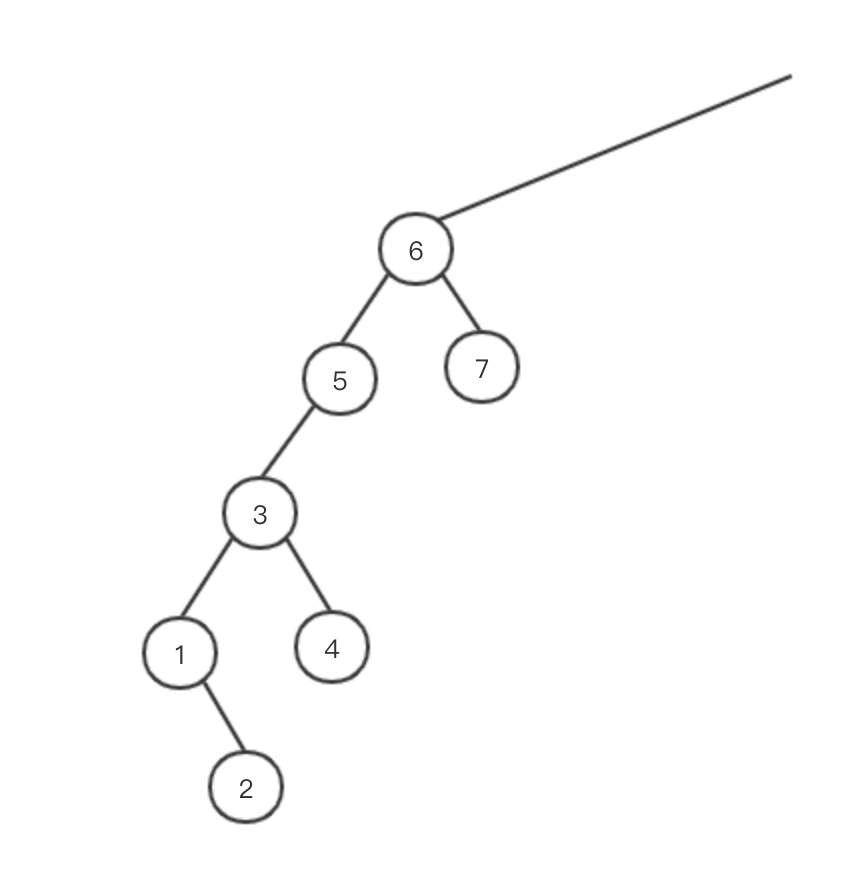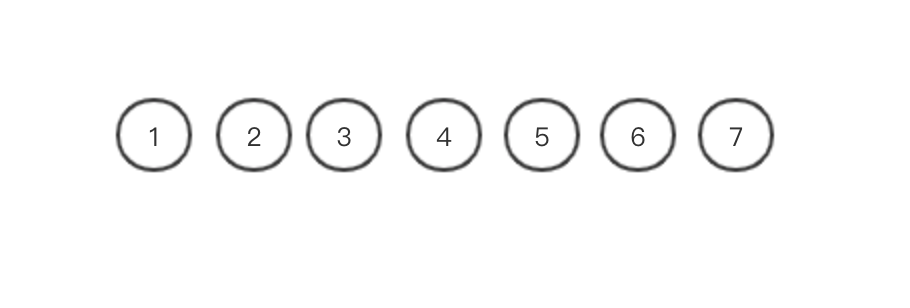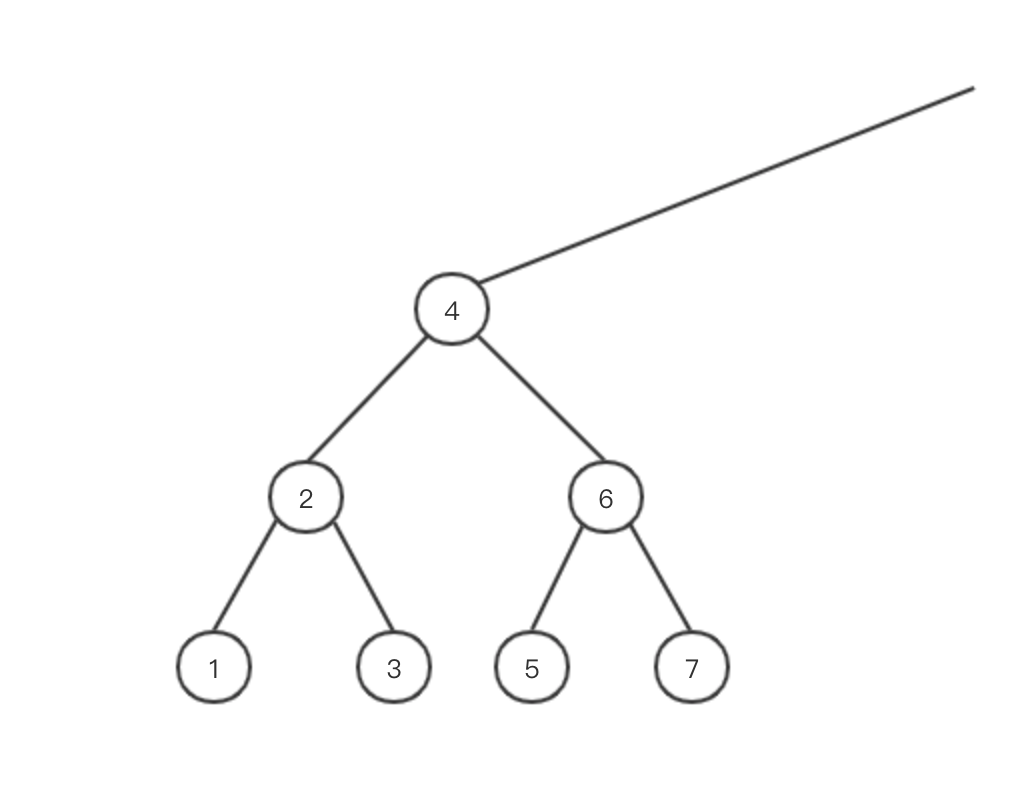Categories

# Scapegoat tree – also a kind of elegant violence

As a binary search tree, then the most important thing is how to keep their balance in order to maintain balance, binary search trees are Eight Immortals recount, such as AVL trees, red-black tree, Treap trees, splay trees, etc., but the original aim, their methods are based on the rotation, and then change the relationship between the nodes.

In particular, some binary search tree implementation is very tedious, like red-black tree, add and delete nodes need to deal with a total of about a dozen cases, finished debug been evaluated days have been dark times.

The scapegoat tree is an unusual tree, when met imbalance will not find ways to adjust the balance, direct violence on her reconstruction.

reconstructionAbove tree sub-tree, is clearly unbalanced, though they did not know on what conditions to determine whether the balance. We directly subtree tree shoot flat, arranged from small to large (in preorder).The middle of the root element as a new element on both sides were as a child. So for her reconstruction is complete, this feels like to be picked up from the middle, as both sides droop down. After the reconstruction of basic binary tree is a full binary tree, and very efficient.So scapegoat tree is how to determine whether a tree needs to balance it. Very simple, each tree will take a balance factor alpha, in the range of between 0.5 to 1. If the total number of nodes in a tree * alpha

Values ​​for alpha, alpha, if smaller, then the more demanding balance, reconstruction will be more times; alpha greater degree balanced tree will be reduced, the number of reconstruction is also reduced. In general, alpha take relatively modest 0.7.

insert

Insertion ordinary binary beginning no difference, the values ​​into the appropriate leaf node, begins to adjust the balance. If the self-inserted node and from the adjustment, the adjustment after completion deeper level sub-tree back up, if lower levels of the tree does not meet the balance, all of the sub-tree still need to be rebuilt, so there are a lot of reconstruction is meaningless of. Therefore, reconstruction should start from the root, first down to determine the need to rebuild. Analyzing all the nodes is not required, only we need to determine the path from the root node to the leaf node to the newly inserted in elapsed.

So long as the occurrence of a reconstruction do not recursively downward, so the insertion of any of a number, reconstruction occurs once at most.

delete

Delete There are many kinds of practices:

1. Each whether to delete a node, will conduct a top-down judgments need to be rebuilt.

2. Each delete a node does not actually delete, mark it just does not participate in search. When the ratio of a sub-tree in the deleted node is greater than a certain value directly reconstruction, this ratio can take direct alpha, it can be freely controlled by us.

3. Each delete a node does not actually delete, mark it just does not participate in search. When an insert causes a trigger is no longer balanced reconstruction, the way will be marked for deletion of nodes move out not participate in the reconstruction.

The second and the third way not very different ways, are idle deleted, the specific use of which way will do.

Code

Implements only the insertion, deletion will follow-up full.

Tree node structure

``````public class ScapegoatTreeNode {
// 以此节点为根的子树的总节点个数
private int size = 1;
private E value;
private ScapegoatTreeNode leftChild;
private ScapegoatTreeNode rightChild;
ScapegoatTreeNode(E value) {
this.value = value;
}

public int getSize() {
return size;
}

public void setSize(int size) {
this.size = size;
}

public E getValue() {
return value;
}

public void setValue(E value) {
this.value = value;
}

public ScapegoatTreeNode getLeftChild() {
return leftChild;
}

public void setLeftChild(ScapegoatTreeNode leftChild) {
this.leftChild = leftChild;
}

public ScapegoatTreeNode getRightChild() {
return rightChild;
}

public void setRightChild(ScapegoatTreeNode rightChild) {
this.rightChild = rightChild;
}
}``````

Insert

``````public class ScapegoatTree> {

private ScapegoatTreeNode root;
private static final double ALPHA_MAX = 1;
private static final double ALPHA_MIN = 0.5;
private double alpha = 0.7;

private List> insertPath = new ArrayList<>();

public ScapegoatTree() {
}

public ScapegoatTree(double alpha) {
if (alpha < 0.5) {
alpha = 0.5;
}
if (alpha > 1) {
alpha = 0.99;
}
this.alpha = alpha;
}

public void insert(E value) {
ScapegoatTreeNode node = new ScapegoatTreeNode<>(value);
if (root == null) {
root = new ScapegoatTreeNode<>(value);
} else {
boolean successfullyInsertion = insertValue(root, node);
if (successfullyInsertion) {
insertPath.forEach(node->node.size++);
}
clearInsertPath();
}
}

private boolean insertValue(ScapegoatTreeNode parent, ScapegoatTreeNode node) {
if (parent == null || node == null) {
return false;
}
int com = node.getValue().compareTo(parent.getValue());
if (com < 0) {
if (parent.getLeftChild() != null) {
return insertValue(parent.getLeftChild(), node);
} else {
parent.setLeftChild(node);
return true;
}
} else if (com > 0) {
if (parent.getRightChild() != null) {
return insertValue(parent.getRightChild(), node);
} else {
parent.setRightChild(node);
return true;
}
}
return false;
}

for (int i = 0; i < insertPath.size(); i++) {
ScapegoatTreeNode node = insertPath.get(i);
int leftChildNodeCount = Optional.ofNullable(node.getLeftChild())
.map(left -> left.size)
.orElse(0);
if (leftChildNodeCount > (int)(node.size * alpha) || leftChildNodeCount < (int)(node.size * (1 - alpha))) {
rebuild(node, i == 0 ? null : insertPath.get(i - 1));
return;
}
}
}

private void rebuild(ScapegoatTreeNode root, ScapegoatTreeNode parent) {
List elements = new ArrayList<>();
inOrderTraversal(root, elements);

ScapegoatTreeNode newRoot = reBuildCore(elements,0, elements.size() - 1);
if (parent == null) {
this.root = newRoot;
} else if (parent.getLeftChild() == root) {
parent.setLeftChild(newRoot);
} else {
parent.setRightChild(newRoot);
}
}

private void inOrderTraversal(ScapegoatTreeNode root, List elements) {
if (root == null) {
return;
}
inOrderTraversal(root.getLeftChild(), elements);
inOrderTraversal(root.getRightChild(), elements);
}

private ScapegoatTreeNode reBuildCore(List elements, int start, int end) {
if (start > end) {
return null;
}
int middle = (int)Math.ceil((start + end) / 2.0);
if (middle >= elements.size()) {
return null;
}

ScapegoatTreeNode root = new ScapegoatTreeNode<>(elements.get(middle));
root.size = end - start + 1;
root.setLeftChild(reBuildCore(elements, start, middle - 1));
root.setRightChild(reBuildCore(elements, middle + 1, end));
return root;
}

private void clearInsertPath() {
insertPath.clear();
}
}``````

Original starting in www.peihuan.net, please indicate the source